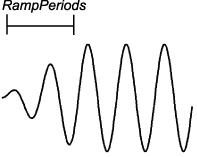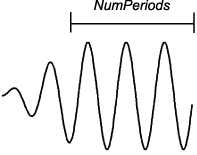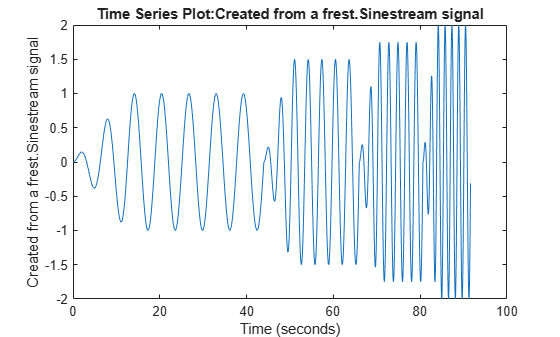# frest.Sinestream

Input signal containing series of sine waves

## Description

Use a `frest.Sinestream` object to represent a sinestream input signal for frequency response estimation. Such a signal consists of sine waves of varying frequencies applied one after another. Each frequency excites the system for a period of time.

Sinestream signals are recommended for most situations. They are especially useful when your system contains strong nonlinearities or you require highly accurate frequency response models. The frequency-response model that results when you use a sinestream input contains all the frequencies in the sinestream signal

You can use a sinestream input signal for estimation at the command line, in the Model Linearizer, or with the Frequency Response Estimator block. The estimation algorithm injects the sinestream signal at the input point you specify for estimation, and measures the response at the output point. For more information, see Sinestream Input Signals.

To view a plot of your input signal, type `plot(input)`. To create a `timeseries` object for your input signal, use the `generateTimeseries` command.

## Creation

You can create a sinestream signal in one of the following ways:

### Syntax

``input = frest.Sinestream(sys)``
``input = frest.Sinestream(Name,Value)``

### Description

example

````input = frest.Sinestream(sys)` creates a signal with a series of sinusoids with properties based on the dynamics of the linear system `sys`. For instance, if you have an exact linearization of your system, you can use it to initialize the parameters.```

example

````input = frest.Sinestream(Name,Value)` creates a signal with a series of sinusoids with properties specified using one or more name-value pairs. Enclose each property name in quotes.```

### Input Arguments

expand all

Linear dynamic system, specified as a SISO `ss`, `tf`, or `zpk` object. You can specify known dynamics or obtain the linear model by linearizing a nonlinear system.

The resulting `frest.Sinestream` object automatically sets the following properties based on the linear system:

• `Frequency` contains the frequencies at which the linear system has interesting dynamics.

• `SettlingPeriods` is the number of periods it takes the system to reach steady state at each frequency in `Frequency`.

• `NumPeriods` is (`3` + `SettlingPeriods`) to ensure that each frequency excites the system at the maximum amplitude for at least three periods.

• For discrete systems only, `SamplesPerPeriod` is set such that all frequencies have the same sample time as the linear system.

The remaining properties use default values.

## Properties

expand all

Signal frequencies, specified as a vector of frequency values in units specified by `FreqUnits`.

Signal amplitude at each frequency, specified as one of the following:

• Scalar — Set all frequencies to same amplitude.

• Vector with length equal to the length of `Frequency` — Set amplitude for each frequency to a different value.

Number of samples per period for each frequency, specified as one of the following:

• Scalar — Use the same number of samples per period for all frequencies.

• Vector with length equal to the length of `Frequency` — Use a different number of samples for each frequency.

Frequency units, specified as one of the following:

• `'rad/s'` — Radians per second

• `'Hz'` — Hertz

Number of periods for ramping up the amplitude of each sine wave to its maximum value, specified as one of the following:

• Scalar — Use the same number of ramping up periods for all frequencies.

• Vector with length equal to the length of `Frequency` — Use a different number of ramping up periods for each frequency.

Use `RampUpPeriods` to specify the number of periods over which to linearly increase the amplitude of each sine wave to its maximum value. Specifying this option ensures a smooth response when your input amplitude changes.`frestimate` discards response data collected during the ramping up periods.

Number of periods each sine wave is at maximum amplitude, specified as one of the following:

• Scalar — Use the same number of periods for all frequencies.

• Vector with length equal to the length of `Frequency` — Use a different number of periods for each frequency.

The specified number of periods includes the settling periods (`SettlingPeriods`) and the periods used for estimation.Number of periods before the system reaches steady state, specified as one of the following:

• Scalar — Use the same number of settling periods for all frequencies.

• Vector with length equal to the length of `Frequency` — Use a different number of settling periods for each frequency.`frestimate` discards response data collected during the settling periods.

Flag to apply frequency-selective FIR filtering of the input signal before estimating it using `frestimate`, specified as one of the following:

• `'on'` — Filter the input signal. When you use filtering, `frestimate` discards response data for one additional period after the settling periods before estimation.

• `'off'` — Do not filter the input signal.

Order in which individual input signal frequencies are injected into your Simulink® model during simulation, specified as one of the following:

• `'Sequential'``frestimate` injects one frequency after the next into your model in a single Simulink simulation using variable sample time. To use this option, your model must use a variable-step solver.

• `'OneAtATime'``frestimate` injects each frequency during a separate Simulink simulation of your model. Before each simulation, `frestimate` initializes your model to the operating point specified for estimation. If you have Parallel Computing Toolbox™ software, you can run these simulations in parallel to speed up estimation. For more information, see Speeding Up Estimation Using Parallel Computing.

## Object Functions

 `frestimate` Frequency response estimation of Simulink models `generateTimeseries` Generate time-domain data for input signal `frest.simCompare` Plot time-domain simulation of nonlinear and linear models `frest.simView` Plot frequency response model in time- and frequency-domain `getSimulationTime` Final time of simulation for frequency response estimation

## Examples

collapse all

Create a sinestream input signal for estimation by specifying the frequencies for the signal. Also, specify the amplitude, the number of ramp-up periods, the number of settling periods, and the total number of periods after the ramp-up.

To specify the frequencies, use a vector of frequencies.

`freqs = linspace(1,4,4);`

To specify the other parameters, use a scalar to use the same parameter value for every frequency. To use different values for each frequency, use a vector of the same length as `freqs`. For this example, use increasing amplitudes at each frequency, but keep the number of ramp-up periods, number of settling periods, and the number of periods after ramp-up constant.

```amps = [1 1.5 1.75 2]; ramp = 2; settle = 3; pds = 5; input = frest.Sinestream('Frequency',freqs,... 'Amplitude',amps,... 'RampPeriods',ramp,... 'SettlingPeriods',settle,... 'NumPeriods',pds);```

Examine the resulting sinestream signal.

`plot(input)`When your sinestream signal covers a wide range of frequencies, it can be inefficient to use the same sample time across all frequencies. For that reason, `frest.Sinestream` by default uses a fixed number of samples at each frequency. You can specify that number with a scalar value, or use a vector to provide a different number of samples at each frequency. (To create a sinestream signal with a fixed sample time across the entire signal, use `frest.createFixedTsSinestream`. This option is useful when the input linearization point for estimation is on a discrete-time signal.)

Create a sinusoidal input signal with the following characteristics:

• 50 frequencies spaced logarithmically between 10 Hz and 1000 Hz

• Amplitude of 1e-3 at all frequencies

• Sampled with a frequency 10 times the frequency of the signal (meaning ten samples per period)

```input = frest.Sinestream('Amplitude',1e-3,... 'Frequency',logspace(1,3,50),... 'SamplesPerPeriod',10,... 'FreqUnits','Hz');```

Create a sinestream input signal based on the dynamics of a linear system. This approach is useful when you are using frequency response estimation to validate the linearization of your model.

```model = 'watertank'; open_system(model) ```For this example, linearize the model at a steady-state operating point to obtain a state-space model you can use to initialize the sinestream signal.

```io(1)=linio('watertank/PID Controller',1,'input'); io(2)=linio('watertank/Water-Tank System',1,'openoutput'); watertank_spec = operspec(model); opOpts = findopOptions('DisplayReport','off'); op = findop(model,watertank_spec,opOpts); sys = linearize(model,op,io); ```

Create the sinestream signal.

```input = frest.Sinestream(sys); ```

`frest.Sinestream` chooses frequencies based on the system dynamics. It also automatically initializes other parameters of the sinestream signal.

```input ```
``` The sinestream input signal: Frequency : [0.0015811;0.0026375;0.0043996;0.007339 ...] (rad/s) Amplitude : 1e-05 SamplesPerPeriod : 40 NumPeriods : [4;4;4;4 ...] RampPeriods : 0 FreqUnits (rad/s,Hz): rad/s SettlingPeriods : [1;1;1;1 ...] ApplyFilteringInFRESTIMATE (on/off) : on SimulationOrder (Sequential/OneAtATime): Sequential ```

You can change properties of the signal using dot notation. For instance, increase the signal amplitude.

```input.Amplitude = 3e-5 ```
``` The sinestream input signal: Frequency : [0.0015811;0.0026375;0.0043996;0.007339 ...] (rad/s) Amplitude : 3e-05 SamplesPerPeriod : 40 NumPeriods : [4;4;4;4 ...] RampPeriods : 0 FreqUnits (rad/s,Hz): rad/s SettlingPeriods : [1;1;1;1 ...] ApplyFilteringInFRESTIMATE (on/off) : on SimulationOrder (Sequential/OneAtATime): Sequential ```

## Alternative Functionality

### Model Linearizer

In the Model Linearizer, to use a sinestream input signal for estimation, on the Estimation tab, select:

• Input Signal > Sinestream when the sample time of the I/Os is continuous.

• Input Signal > Fixed Sample Time Sinestream when the sample time of the I/Os is discrete.